Search

How Can 0.999... = 1?

There are many different proofs of the fact that "0.9999..." does indeed equal 1. So why does this question keep coming up?

Students don't generally argue with "0.3333..." being equal to 1/3, but then, one-third is a fraction. Maybe it's just that it "feels" "wrong" that something as nice and neat and well-behaved as the number "1" could also be written in such a messy form as "0.9999..." Whatever the reason, many students (me included) have, at one time or another, felt uncomfortable with this equality.

One of the major sticking points seems to be notational, so let me get that out of the way first: When I say "0.9999...", I don't mean "0.9" or "0.99" or "0.9999" or "0.999" followed by some large but finite (limited) number of 9's". The ellipsis (the "dot, dot, dot" after the last 9) means "goes on forever in like manner". In other words, "0.9999..." never ends. There will always be another "9" to tack onto the end of 0.9999.... So don't object to 0.9999... = 1 on the basis of "however far you go out, you still won't be equal to 1", because there is no "however far" to "go out" to; you can always go further.

"But", some say, "there will always be a difference between 0.9999... and 1." Well, sort of. Yes, at any given stop, at any given stage of the expansion, for any given finite number of 9s, there will be a difference between 0.999...9 and 1. That is, if you do the subtraction, 1  0.999...9 will not equal zero. But the point of the "dot, dot, dot" is that there is no end; 0.9999... is inifinte. There is no "last" digit. So the "there's always a difference" argument betrays a lack of understanding of the infinite. (That's not a "criticism", per se; infinity is a messy topic.)

Proof by geometric series

The number "0.9999..." can be "expanded" as:

0.9999... = 0.9 + 0.09 + 0.009 + 0.0009 + ...

In other words, each term in this endless summation will have a "9" preceded by some number of zeroes. This may also be written as: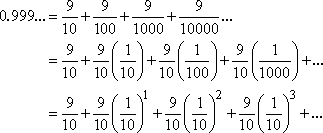That is, this is an infinite geometric series with first term a =  9/10 and common ratio r =  1/10. Since the size of the common ratio r is less than 1, we can use the infinite-sum formula to find the value: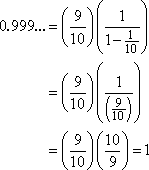So the formula proves that 0.9999... = 1.

Note: Technically, the above proof requires that some fairly advanced concepts be taken on faith. If you study "foundations" or mathematical philosophy (way after calculus), you may encounter the requisite theoretical constructs.   Copyright © Elizabeth Stapel 2006-2011 All Rights Reserved

Other pre-calculus arguments

Argument from precedence: If you haven't already learned that 1/3 = 0.333... in decimal form, you can prove this easily by doing the long division: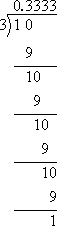So 1/3 + 1/3 + 1/3 = 3( 1/3 ) = 1. Reasonably then, 0.333... + 0.333... + 0.333... = 3(0.333...) should also equal 1. But 3(0.333...) = 0.999.... Then 0.999... must equal 1.

Argument from arithmetic: When you subtract a number from itself, the result is zero. For instance, 4  4 = 0. So what is the result when you subtract 0.999... from 1? For shorter subtractions, you get: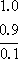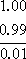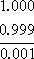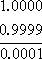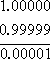Then what about 1.000...  0.999...? You'll get an infinite string of zeroes. "But," you ask, "what about that '1' at the end?" Ah, but 0.999... is an infinite decimal; there is no "end", and thus there is no "1 at the end". The zeroes go on forever. And 0.000... = 0.

Then 1  0.999... = 0.000... = 0, and 1 = 0.999....

Argument from philosophy: If two numbers are different, then you can fit another number between them, such as their average. But what number could you possibly fit between 0.999... and 1.000...?

Argument from algebra: The expression 0.999... is some number; it has some value. Call this numerical value "x", so 0.999... = x. Multiply this equation by ten:

x = 0.999...
10x = 9.999...

Subtract the former from the latter: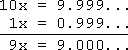Solving, we get x = 1, so 0.999... = 1.

"But," you ask, "when you multiply by ten, that puts a zero at the end, doesn't it?" For finite expansions, certainly; but 0.999... is infinite. There is no "end" after which to put that zero.

Argument from semantics: A common objection is that, while 0.999... "gets arbitrarily close" to 1, it is never actually equal to 1. But what is meant by "gets arbitrarily close"? It's not like the number is moving at all; it is what it is, and it just sits there, looking at you. It doesn't "come" or "go" or "move" or "get close" to anything.

On the other hand, the terms of the associated sequence, 0.9, 0.99, 0.999, 0.9999, ..., do "get arbitrarily close" to 1, in the sense that, for each term in the progression, the difference between that term and 1 gets smaller and smaller. No matter how small you want that difference to be, I can find a term where the difference is even smaller.

This "getting arbitrarily close" process refers to something called "limits". You'll learn about limits later, probably in calculus. And, according to limit theory, "getting arbitrarily close" means that they're equal: 0.999... does indeed equal 1.

Note regarding all of the above: To a certain extent, each of these arguments depends on a basic foundational doctrine of mathematics called "The Axiom of Choice". A discussion of the Axiom of Choice is well beyond anything we could cover here, and is something that most mathematicians simply take on faith.